# 使用 COMSOL Multiphysics? 模擬 COVID-19 的傳播

2020年 4月 7日

### COVID-19 的數學模型

S?=易感者

E?=潛伏者

I?=感染者

R?=康復并具有免疫力的人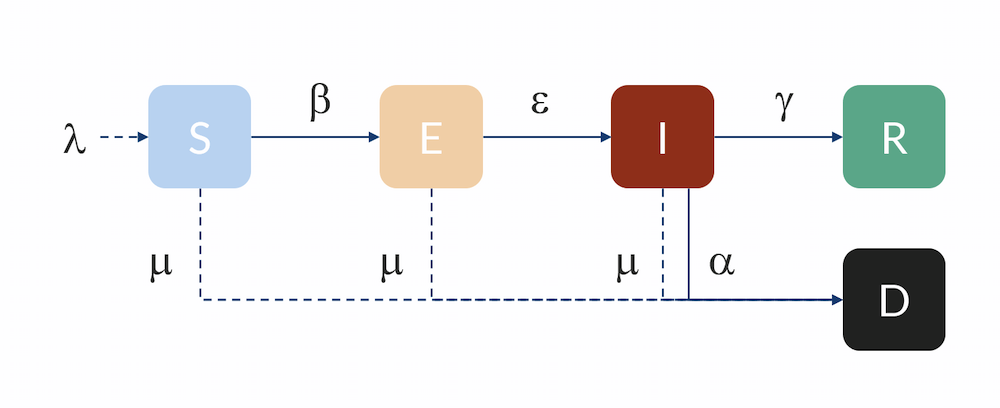(1)

${r_{nE}} = \frac{\beta }{N}SI$

β（單位：1人 /天）與基本傳染數 R?0和感染者具有傳染性的平均天數（在被隔離或自我隔離之前）有關，nid

(2)

$\beta = \frac{{{R_0}}}{{{n_{id}}}}$

R?0??稱為基本傳染數（無量綱），它描述了感染者在康復之前與易感人的每次接觸（當人群中完全沒有免疫力時）的疾病傳播。任何緩解或隔離措施都旨在通過減少傳染率 β 或及時隔離感染者，來降低傳染數。

(3)

$\frac{{dS}}{{dt}} = – \frac{\beta }{N}SI$

(4)

$\frac{{dE}}{{dt}} = \frac{\beta }{N}SI – \varepsilon E$

(5)

$\gamma = \frac{1}{{{n_{id}}}}$

(6)

$\frac{{dI}}{{dt}} = \varepsilon E – \gamma I – \alpha I$

(7)

$\frac{{dR}}{{dt}} = \gamma I$

(8)

$\frac{{dD}}{{dt}} = \alpha I$

### 展平曲線

N?= 100 萬個人

R?0?= 2.25，基本傳染數

??ID?=5 天

S?0??=?N?–??I?0，易感人群

I?0??= 10 個感染者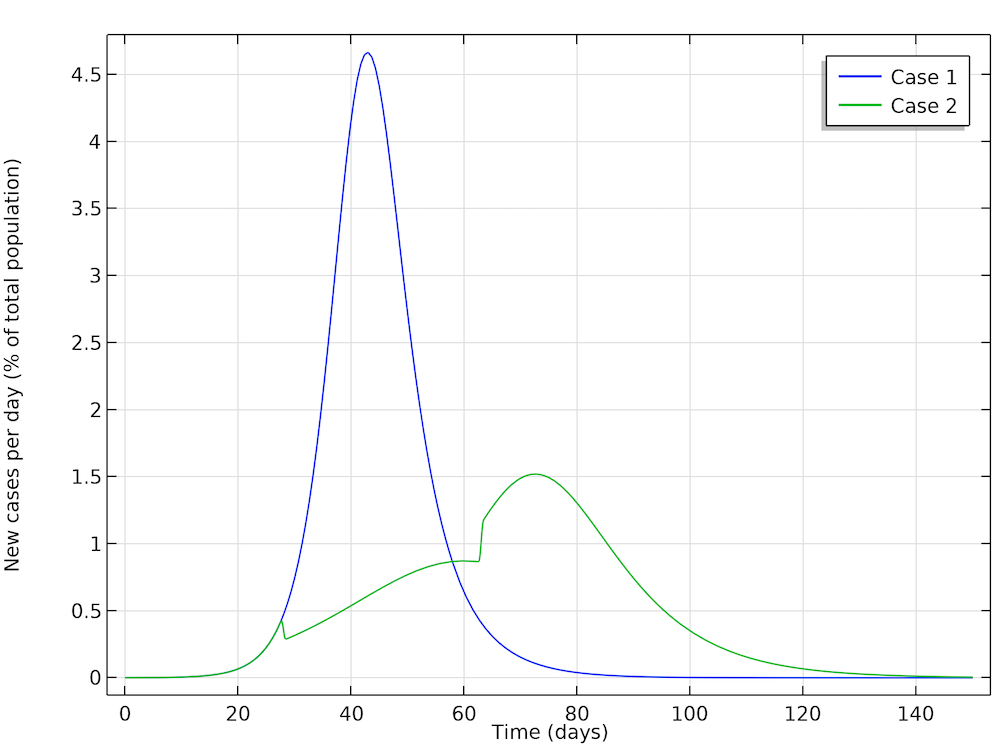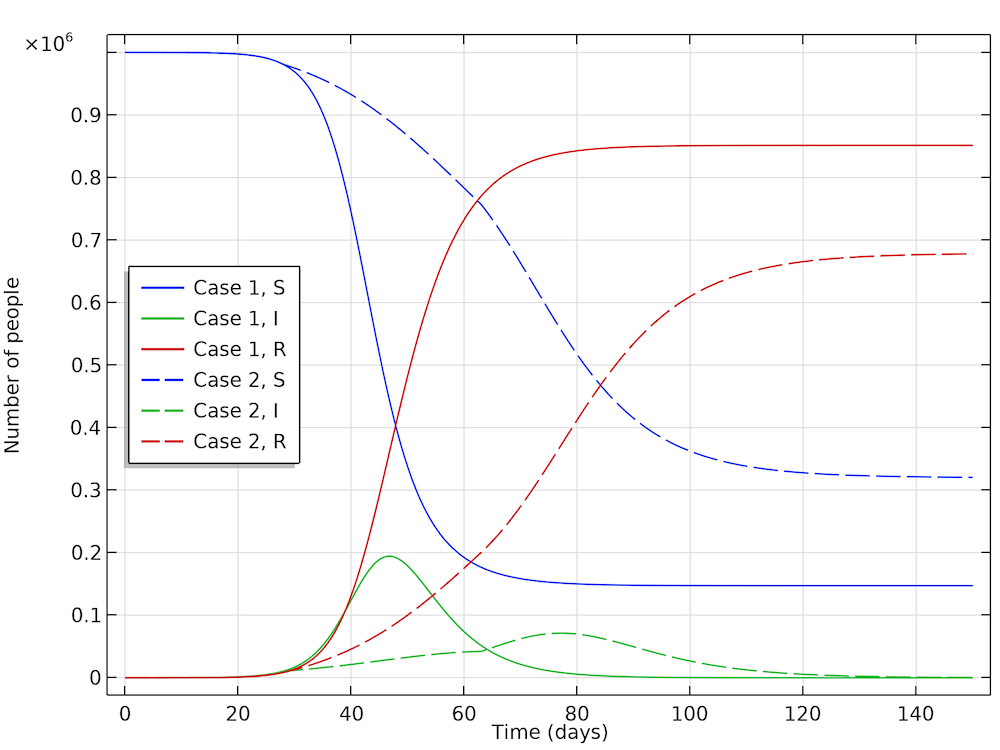### 考慮不同人群分布的流行病發展過程計算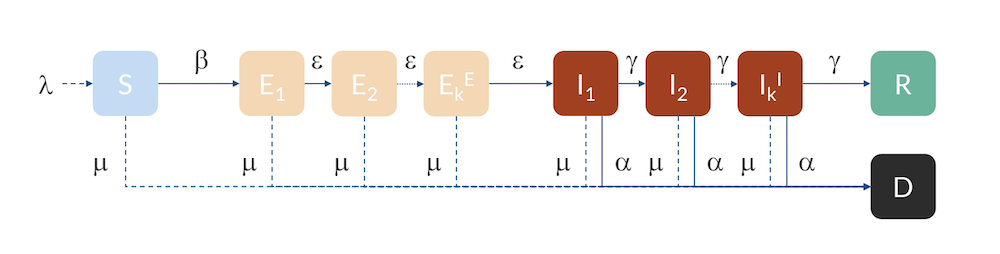### COVID-19在中國湖北的傳播模擬

2019年1月22日，當局實施了封鎖措施，并在該月底進行了測量，結果顯示基本傳染數明顯下降（參考文獻5） 。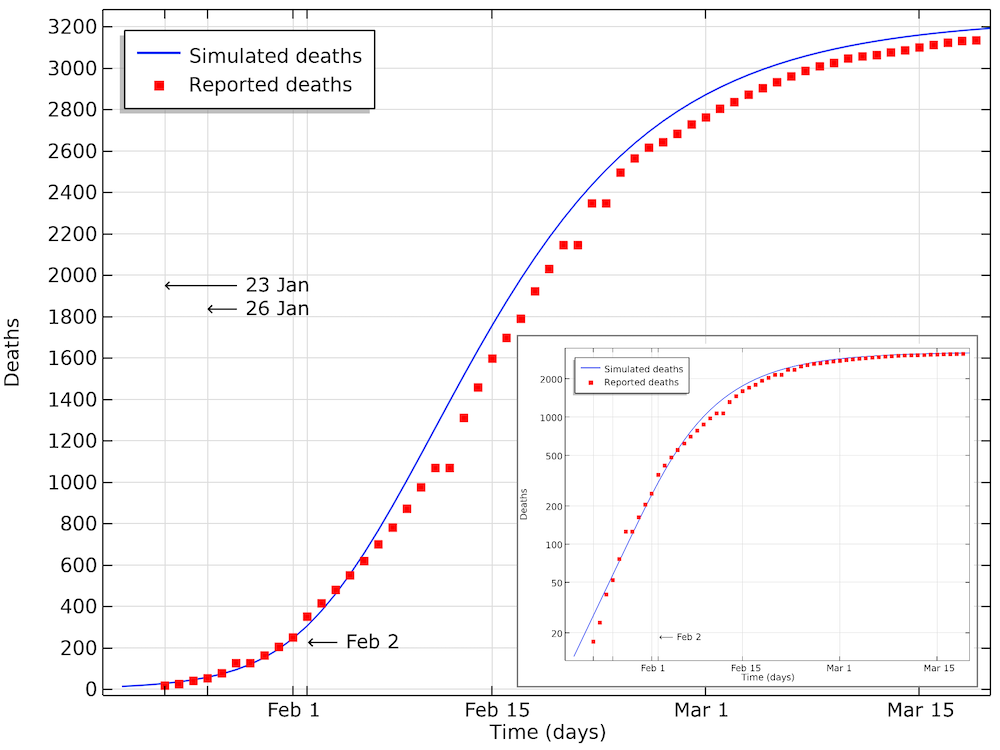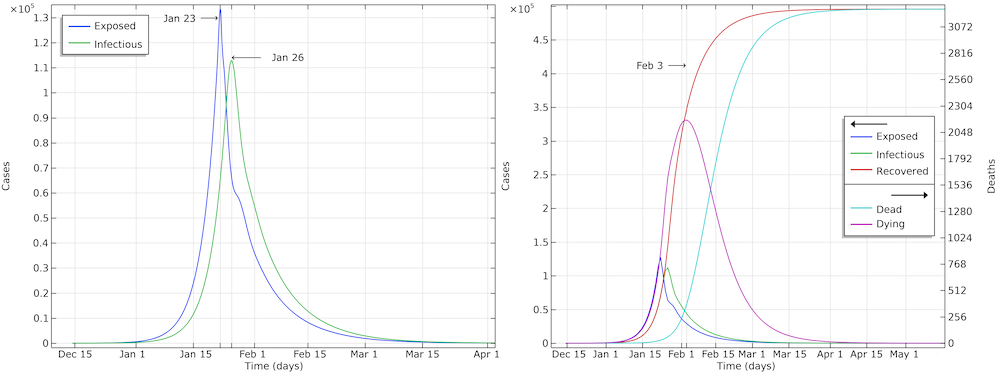### 瑞典：保持社交距離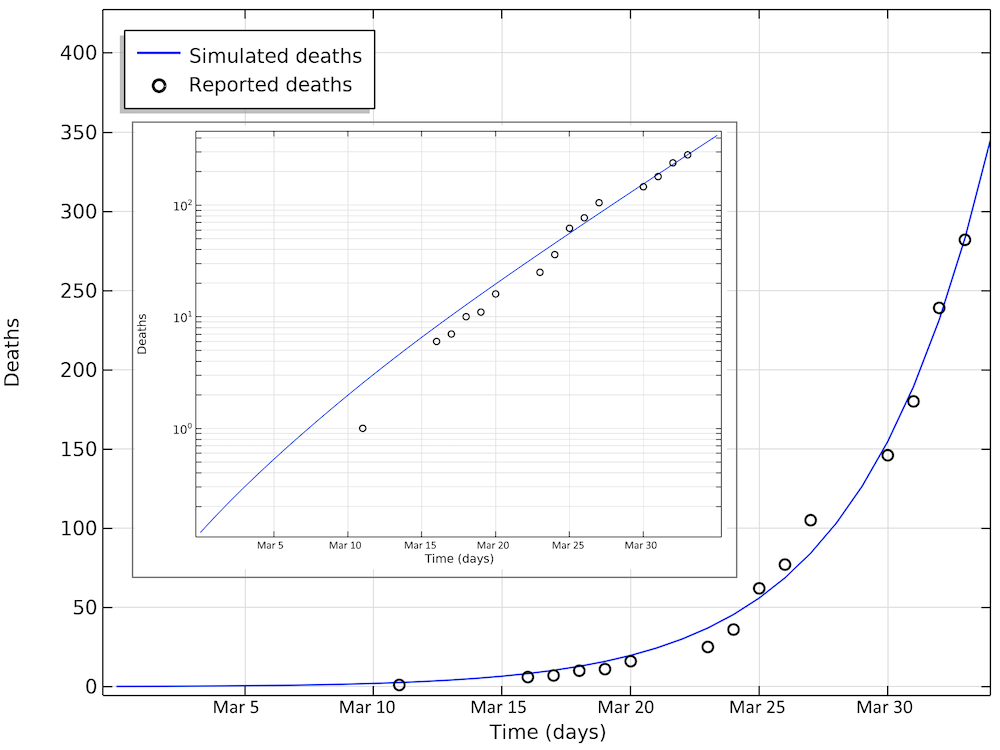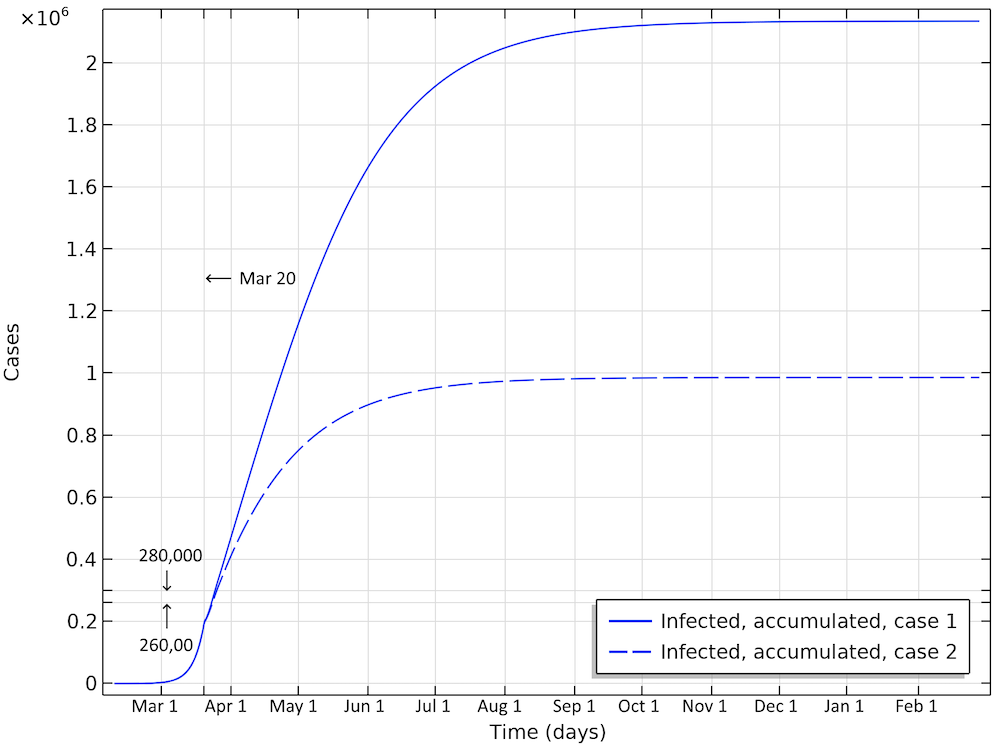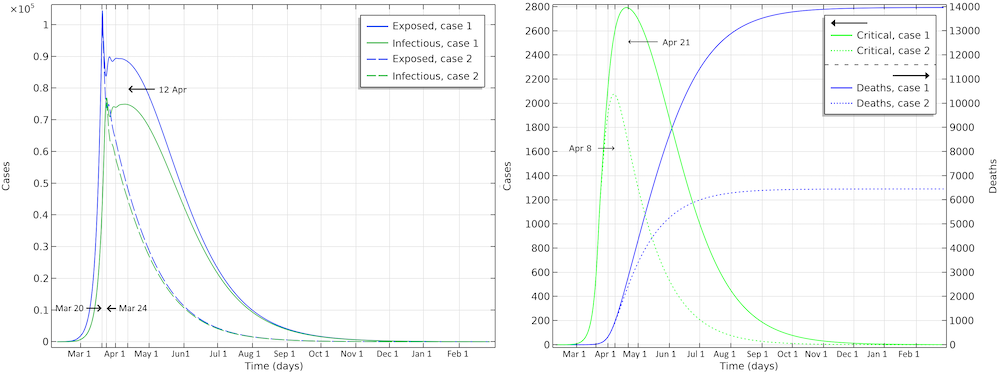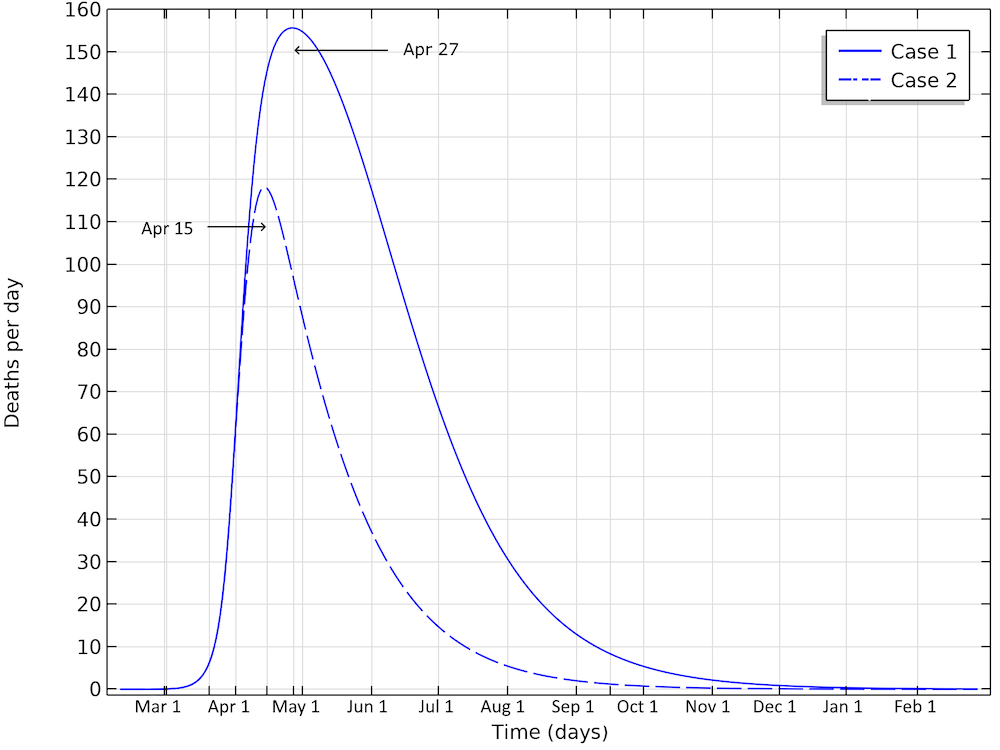### 美國：大規模隔離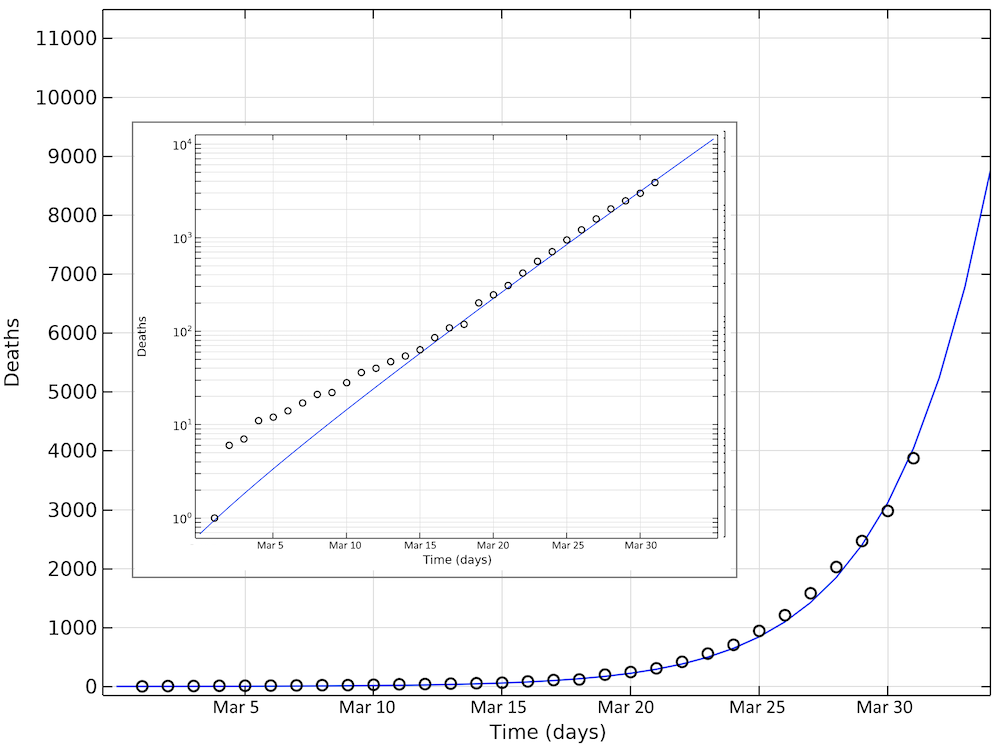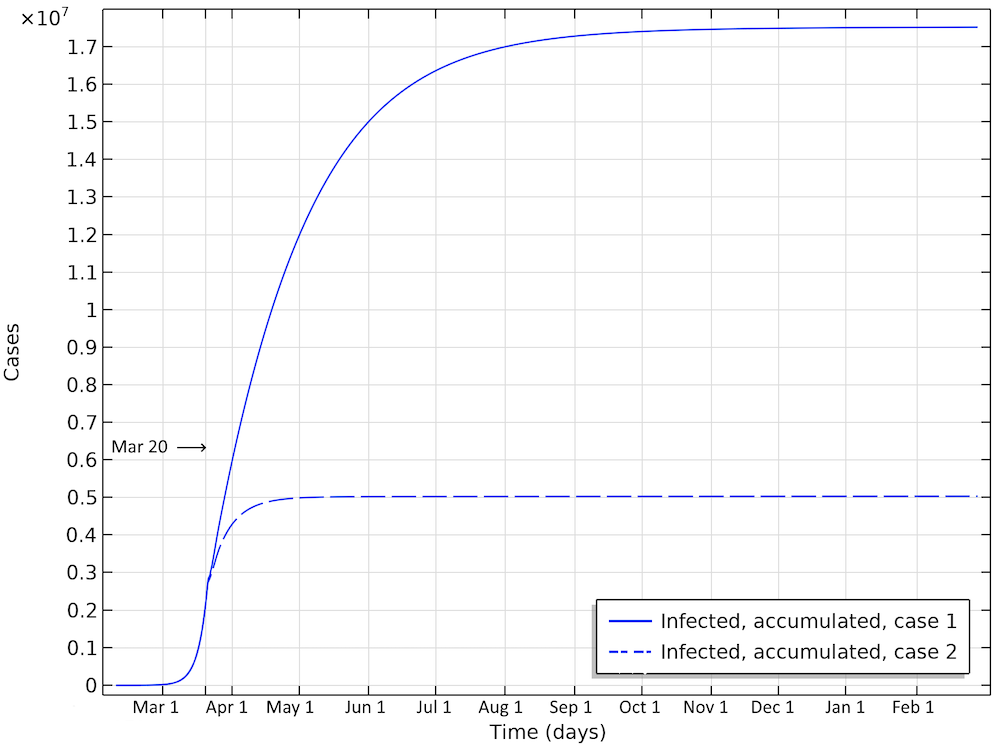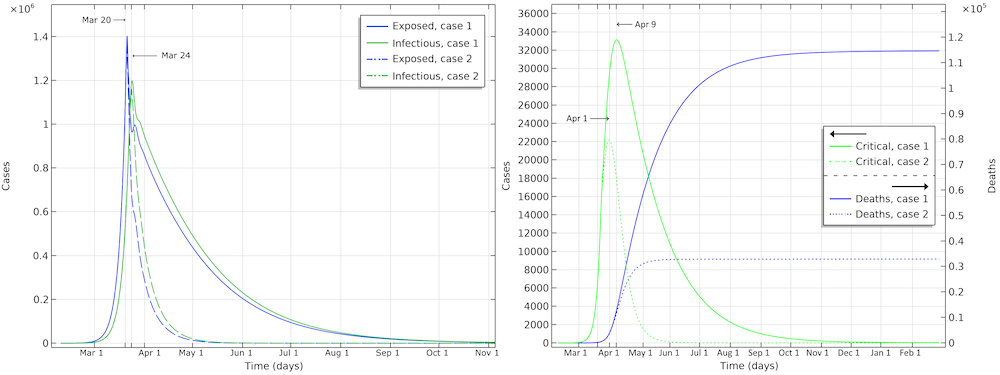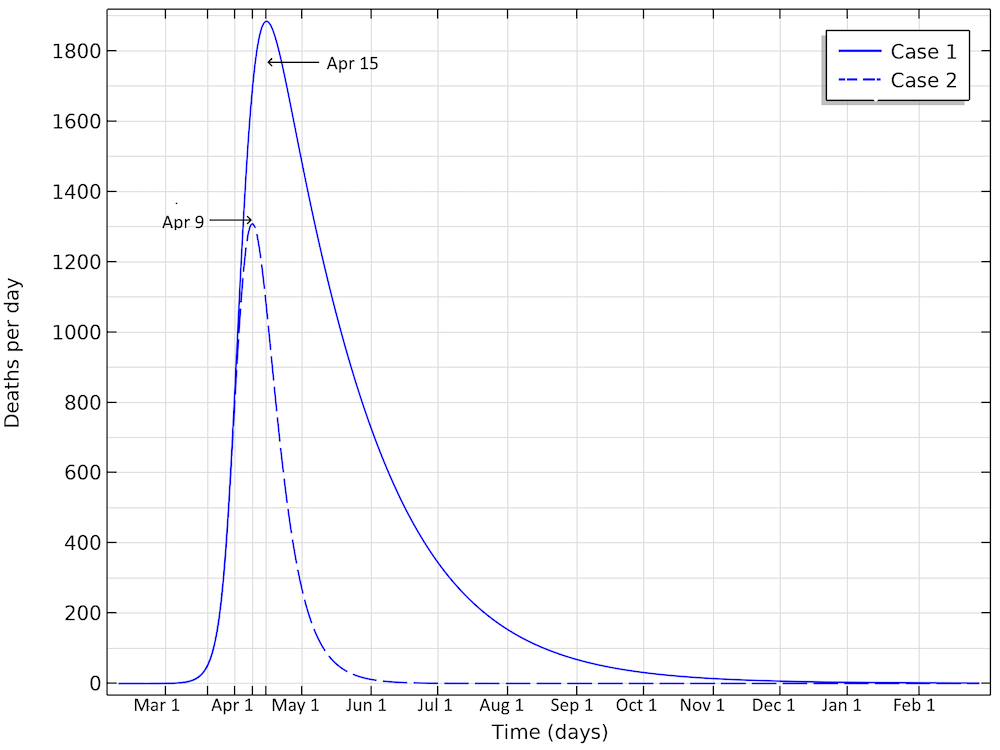### 下載模型和應用文件

1. H. Weiss, “The SIR model and the Foundations of Public Health”, MATerials MATemàtics, vol. 2013, no. 3, pp. 1–17, 2013.
2. M. H?hle, “Flatten the COVID-19 curve”, Theory meets practice…, 2020.
3. W.M. Getz and E.R. Dougherty, “Discrete Stochastic Analogs of Erlang Epidemic Models”, Journal of Biological Dynamics, vol. 12, pp.16–38, 2018.
4. R. Verity, L.C. Okell, I. Dorigatti, P. Winskill, C. Whittaker, et al., “Estimates of the severity of coronavirus disease 2019: a model-based analysis”, The Lancet Infectious Diseases, 2020.
5. A.J. Kucharski, T.W. Russell, C. Diamond, Y. Liu, J. Edmunds, S. Funk, R.M. Eggo, “Early dynamics of transmission and control of COVID-19: a mathematical modelling study”, The Lancet Infectious Diseases, 2020.
6. R. Li, S. Pei, B. Chen, et at., “Substantial undocumented infection facilitates the rapid dissemination of novel coronavirus (SARS-CoV2)”, Science, 2020.
7. Y. Liu, A.A. Gayle, A. Wilder-Smith, and J. Rockl?v, “The reproductive number of COVID-19 is higher compared to SARS coronavirus”, Journal of Travel Medicine, vol. 27, no. 2, 2020.